# 7. (20 pts) Acetate buffers are frequently used in biochemistry when working with proteins. 500 mL...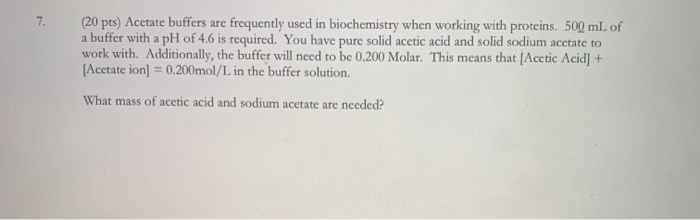7. (20 pts) Acetate buffers are frequently used in biochemistry when working with proteins. 500 mL of a buffer with a pH of 4.6 is required. You have pure solid acetic acid and solid sodium acetate to work with. Additionally, the buffer will need to be 0.200 Molar. This means that (Acetic Acid) + [Acetate ion] = 0.200mol/L in the buffer solution. What mass of acetic acid and sodium acetate are needed?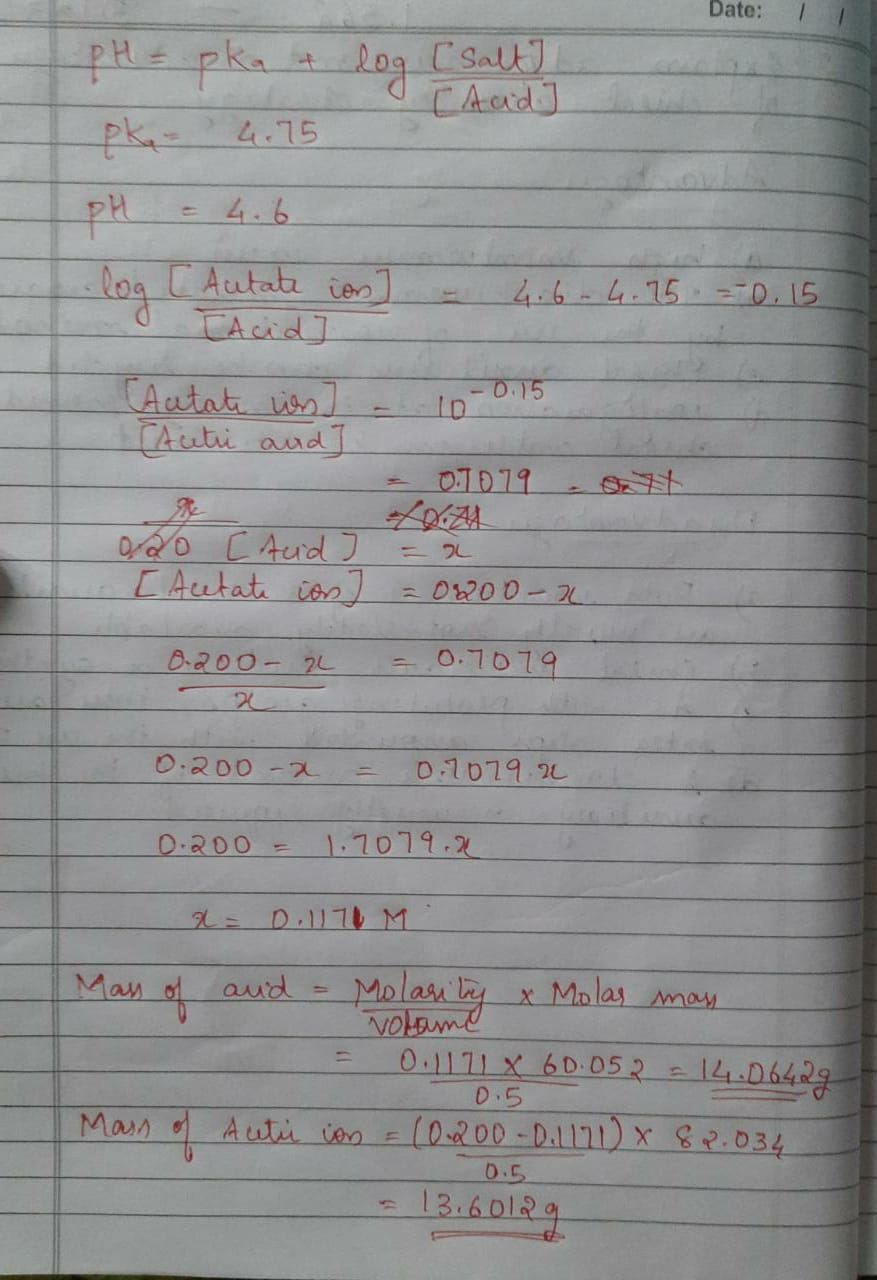##### Add Answer of: 7. (20 pts) Acetate buffers are frequently used in biochemistry when working with proteins. 500 mL...
Similar Homework Help Questions
• ### D. Buffers 1. A buffer solution was made by dissolving 10.0 grams of sodium acetate in...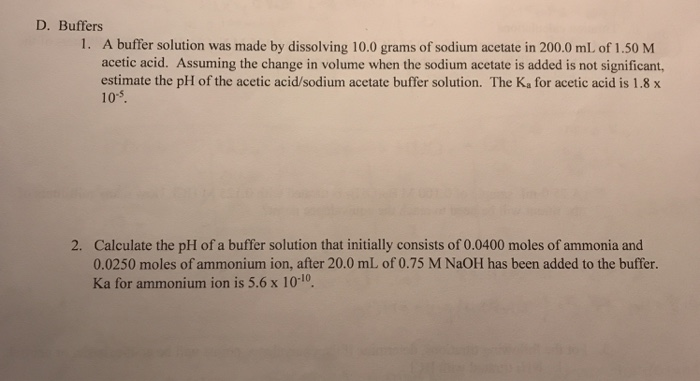D. Buffers 1. A buffer solution was made by dissolving 10.0 grams of sodium acetate in 200.0 mL of 1.50 M acetic acid. Assuming the change in volume when the sodium acetate is added is not significant, estimate the pH of the acetic acid/sodium acetate buffer solution. The K, for acetic acid is 1.8 x 105. 2. Calculate the pH of a buffer solution that initially consists of 0.0400 moles of ammonia and 0.0250 moles of ammonium ion, after 20.0...

• ### 7. Acetate buffers are used in biochemical studies of enzymes and other chemical components of cells...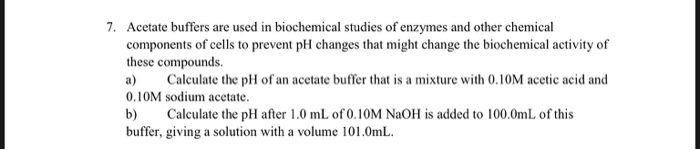7. Acetate buffers are used in biochemical studies of enzymes and other chemical components of cells to prevent pH changes that might change the biochemical activity of these compounds. a) Calculate the pH of an acetate buffer that is a mixture with 0.10M acetic acid and 0.10M sodium acetate. b) Calculate the pH after 1.0 mL of 0.10M NaOH is added to 100.0mL of this buffer, giving a solution with a volume 101.0mL.

• ### You are asked to prepare 500 mL 0.300 M acetate buffer at pH 4.90 using only...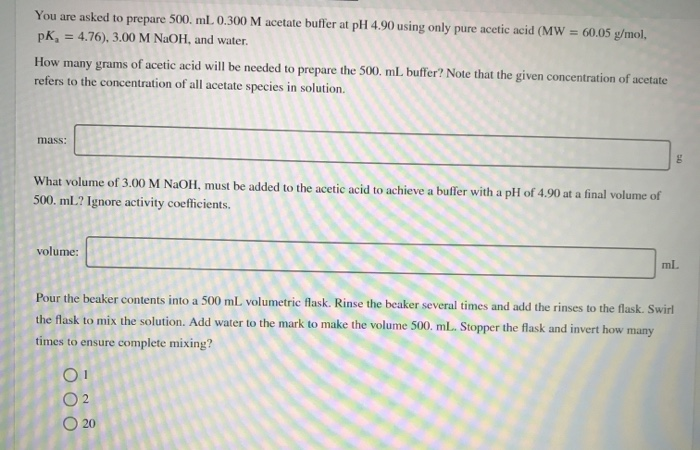You are asked to prepare 500 mL 0.300 M acetate buffer at pH 4.90 using only pure acetic acid (MW = 60.05 g/mol pk, = 4.76), 3.00 M NaOH, and water. How many grams of acetic acid will be needed to prepare the 500 mL buffer? Note that the given concentration of acetate refers to the concentration of all acetate species in solution. mass: What volume of 3.00 M NaOH, must be added to the acetic acid to achieve a...

• ### You are asked to prepare 500. mL 0.150 M acetate buffer at pH 5.10 using only...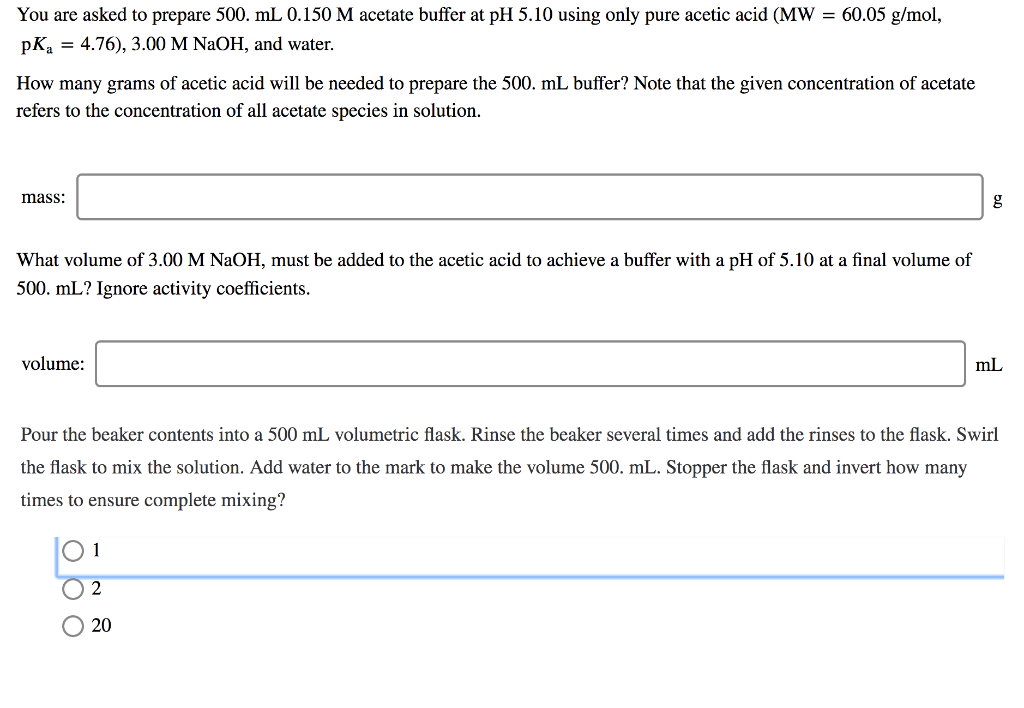You are asked to prepare 500. mL 0.150 M acetate buffer at pH 5.10 using only pure acetic acid (MW = 60.05 g/mol, pka = 4.76), 3.00 M NaOH, and water. How many grams of acetic acid will be needed to prepare the 500. mL buffer? Note that the given concentration of acetate refers to the concentration of all acetate species in solution. mass: DD What volume of 3.00 M NaOH, must be added to the acetic acid to achieve...

• ### Part 1: Buffers Considering the acetic acid sodium acetate buffer, complete the table below NaOH added/mL....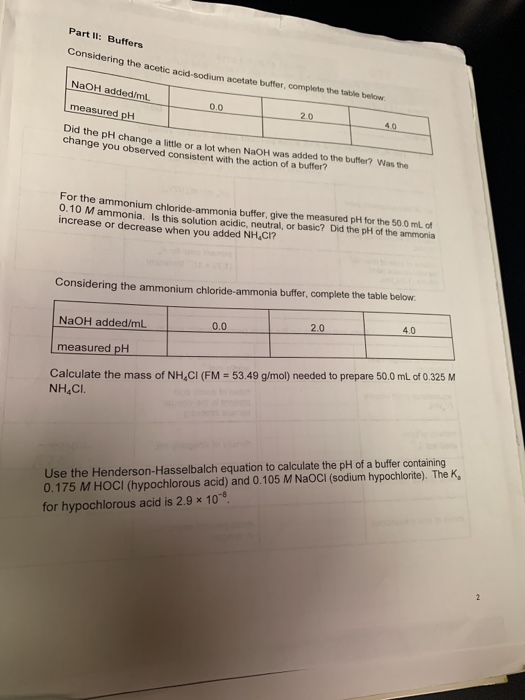Part 1: Buffers Considering the acetic acid sodium acetate buffer, complete the table below NaOH added/mL. measured pH 0.0 20 Did the pH change a little or a lot when NaOH was added to the butter? Was the change you observed consistent with the action of a butter? For the ammonium chloride-ammonia buffer give the measured pH for the 50.0 mL of 0.10 Mammonia. Is this solution acidic, neutral, or basic? Did the pH of the ammonia increase or decrease...

• ### Table 3: Sodium Acetate Data Sodium Acetate (g) Molarity of Sodium Acetate (Step 7) 4.0 g...

Table 3: Sodium Acetate Data Sodium Acetate (g) Molarity of Sodium Acetate (Step 7) 4.0 g 0.4876 M Table 4: Buffer Solutions and pH Readings for Beakers A, B, C, D, and E Buffer mL of Acetic Acid mL of Sodium Acetate pH measured A 5 5 4.3 B 5 1 3.6 C 10 1 3.4 D 1 10 5.3 E 1 5 5.0 Post-Lab Questions What are the calculated pH values for the buffers (A,B,C,D and E) that you...

• ### please answer the questions thank you Name: Lab Section: Date: Experiment VII: Buffers Review Questions (23...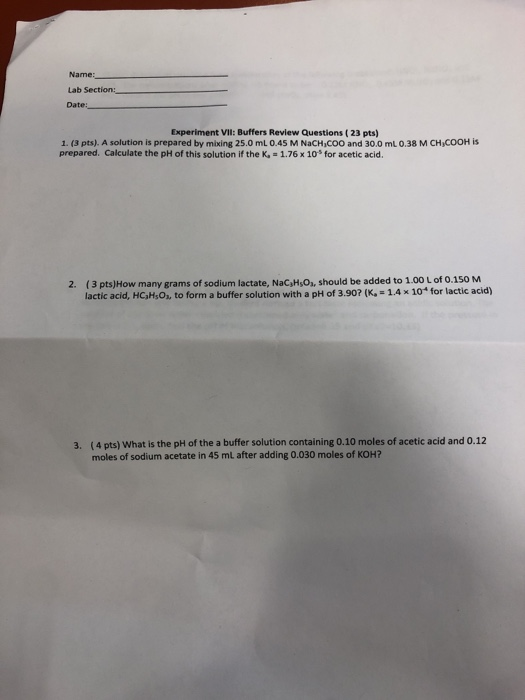please answer the questions thank you Name: Lab Section: Date: Experiment VII: Buffers Review Questions (23 pts) 1. (3 pts). A solution is prepared by mixing 25.0 mL 0.45 M NaCH,coo and 30.0 mL 0.38 M CH,COOH is prepared. Calculate the pH of this solution if the K, 1.76 x 10 for acetic acid. 2. (3 pts)How many grams of sodium lactate, NaCsHsOs, should be added to 1.00 L of 0.150 M lactic acid, HCsHsOs, to form a buffer solution...

• ### According to the Henderson-Hasselbalch equation, how many grams of sodium acetate will you need to add to 125 mL of 0.100 M acetic acid to make a pH=4.74 buffer

a. According to the Henderson-Hasselbalch equation, how many grams of sodium acetate will you need to add to 125 mL of 0.100 M acetic acid to make a pH=4.74 buffer? b. What is the actual pH of the resulting buffer when you add that many grams of sodium acetate to 125 mL of 0.100 M acetic acid? c. If I want to make the buffer by adding 0.100 M NaOH instead of sodium acetate, how many milliliters of NaOH are...

• ### You are asked to prepare 500. mL of a 0.100 M acetate buffer at pH 5.00 using only pure acetic acid (MW=60.05 g/mol,...

You are asked to prepare 500. mL of a 0.100 M acetate buffer at pH 5.00 using only pure acetic acid (MW=60.05 g/mol, pKa=4.76), 3.00 M NaOH, and water. Answer the following questions regarding the preparation of the buffer. 1. How many grams of acetic acid will you need to prepare the 500 mL buffer? Note that the given concentration of acetate refers to the concentration of all acetate species in solution. 2. What volume of 3.00 M NaOH must...

• ### 2. A biochemist wants to make 2.0 L of a 0.4 M acetic acid/acetate buffer with...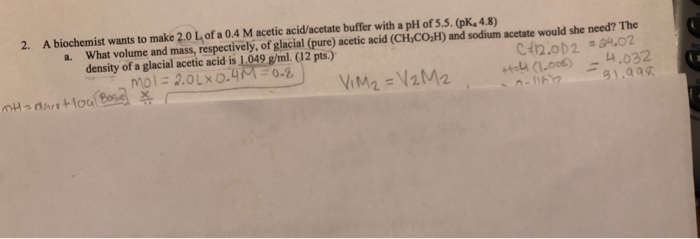2. A biochemist wants to make 2.0 L of a 0.4 M acetic acid/acetate buffer with a pH of 5.5. (pK, 4.8) a. What volume and mass, respectively, of glacial (pure) acetic acid (CH,CO,H) and sodium acetate would she need? The density of a glacial acetic acid is 1.049 g/ml. (12 pts.) mol = 2.0L 0.4M=0.8. C112.002 = 34.02 Marot loa Bose X VIM2 = V2 M2 +24 (1.008) - 4.032 noliny 31.908 TH

Free Homework App